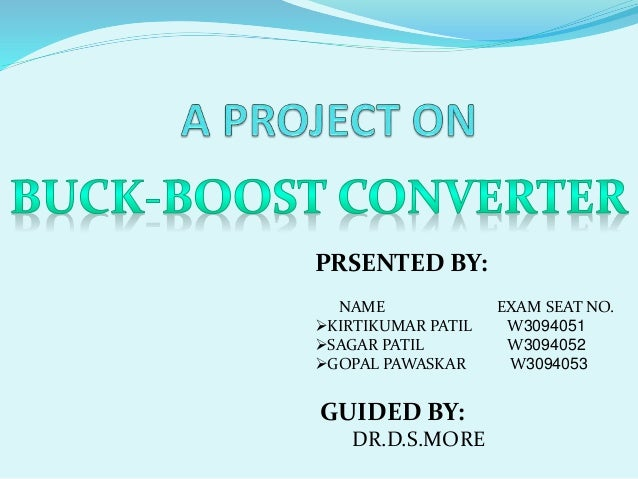Successfully reported this slideshow.
We use your LinkedIn profile and activity data to personalize ads and to show you more relevant ads. You can change your ad preferences anytime.Upcoming SlideShare
×

# Buck-Boost Converter

10,231 views

Published on

It’s a power electronics project. It is able to give output voltage(DC) more and less than input voltage as per requirement.
We can generate variable DC voltage which is less than input, but, the special things about this converter is, it has capability to produce variable DC voltage as high as twice the input voltage.
We have specially designed and manufactured inductor for this project.

Published in: Engineering
• Full Name
Comment goes here.

Are you sure you want to Yes No
Your message goes here• Be the first to comment

### Buck-Boost Converter

1. 1. PRSENTED BY: NAME EXAM SEAT NO. KIRTIKUMAR PATIL W3094051 SAGAR PATIL W3094052 GOPAL PAWASKAR W3094053 GUIDED BY: DR.D.S.MORE
2. 2. INTRODUCTION  Buck-boost converter operates on dc supply  Buck operation  Boost operation  Duty ratio of control signal defines its operation
3. 3. CONTROL CIRCUIT
4. 4. OPERATION OF CONTROL CIRCUIT  To drive the mosfet op-amp based circuit is designed  Op-amp(A) is used as an integrator  Op-amp(B) and op-amp(c) is used as a comparator  Transistor 2N5294 is used as a switch to provide control signal  Frequency and duty ratio of output signal can be controlled by using potentiometer in this circuit
5. 5.  Output waveform of Op-amp(A)  Output waveform of Op-amp(B)  Output waveform of control circuit
6. 6. POWER CIRCUIT
7. 7. OPERATION OF POWER CIRCUIT  Step 1: when mosfet is ON  Voltage across inductor L is equal to V (source voltage)
8. 8.  Step 2: when mosfet is OFF  Voltage across inductor L is equal to Vo (in reverse direction)  Here output voltage developed across load is Vo
9. 9.  Step 3: When mosfet is ON in next cycle Load is isolated from voltage source In this case charged capacitor across load will maitain voltage across load
10. 10. DUTY RATIO  Duty ratio(D) is defined as Ton/T here, Ton=ON time T=time period of gate signal  D=Ton/T Ton=DT T=Ton+Toff Toff=T-Ton Toff=(1-D)T
11. 11. VOLTAGE ACROSS INDUCTOR  When mosfet is ON, voltage across inductor is equal to source voltage(V).  When mosfet is OFF, voltage across inductor is equal to output voltage(Vo)  As average voltage across inductor is zero Hence, V*D*T=Vo*(1-D)*T Vo=V* D 1-D
12. 12. Vo=V* D 1-D  This equation shows that output voltage depends on duty ratio(D)  For buck operation D=0 to 0.5  For boost operation D=0.5 to 1
13. 13. APPLICATIONS  Used in many industrial applications such as subway cars, trolley buses, battery operated vehicles, battery charging etc.  To provide smooth control on output, high efficiency, fast response and regeneration.  To provide efficient control on dc motor operation  To provide long life and less maintenance due to absence of moving parts.